SBAS799 February   2017

PRODUCTION DATA.

1. Features
2. Applications
3. Description
4. Revision History
5. Device Comparison Table
6. Pin Configurations and Functions
7. Specifications
8. Detailed Description
1. 8.1 Overview
2. 8.2 Functional Block Diagram
3. 8.3 Feature Description
4. 8.4 Device Functional Modes
9. Application and Implementation
1. 9.1 Application Information
2. 9.2 Typical Applications
1. 9.2.1 Traction Inverter Application
2. 9.2.2 Isolated Voltage Sensing
3. 9.2.3 Do's and Don'ts
10. 10Power-Supply Recommendations
11. 11Layout
12. 12Device and Documentation Support
13. 13Mechanical, Packaging, and Orderable Information

• DW|16
• DW|16

## 9 Application and Implementation

NOTE

Information in the following applications sections is not part of the TI component specification, and TI does not warrant its accuracy or completeness. TI’s customers are responsible for determining suitability of components for their purposes. Customers should validate and test their design implementation to confirm system functionality.

### 9.1 Application Information

#### 9.1.1 Digital Filter Usage

The modulator generates a bit stream that is processed by a digital filter to obtain a digital word similar to a conversion result of a conventional analog-to-digital converter (ADC). A very simple filter, built with minimal effort and hardware, is a sinc3-type filter, as shown in Equation 2:

Equation 2.This filter provides the best output performance at the lowest hardware size (count of digital gates) for a second-order modulator. All the characterization in this document is also done with a sinc3 filter with an oversampling ratio (OSR) of 256 and an output word duration of 16 bits.

The effective number of bits (ENOB) is often used to compare the performance of ADCs and ΔΣ modulators. Figure 53 shows the ENOB of the AMC1304-Q1 with different oversampling ratios. In this document, this number is calculated from the SNR by using Equation 3:

Equation 3.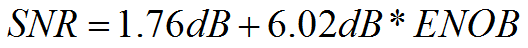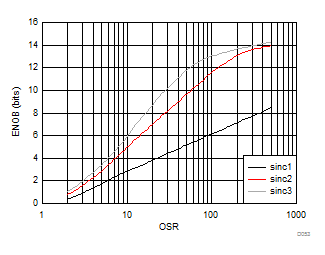Figure 53. Measured Effective Number of Bits versus Oversampling Ratio

An example code for implementing a sinc3 filter in an FPGA is discussed in the Combining ADS1202 with FPGA Digital Filter for Current Measurement in Motor Control Applications application note (SBAA094), available for download at www.ti.com.

### 9.2 Typical Applications

#### 9.2.1 Traction Inverter Application

Isolated ΔΣ modulators are being widely used in new-generation traction inverter designs because of their high ac and dc performance. Traction inverters are critical parts of electrical and hybrid electrical vehicles. The input structure of the AMC1304-Q1 is optimized for use with low-impedance shunt resistors and is therefore tailored for isolated current sensing using shunts.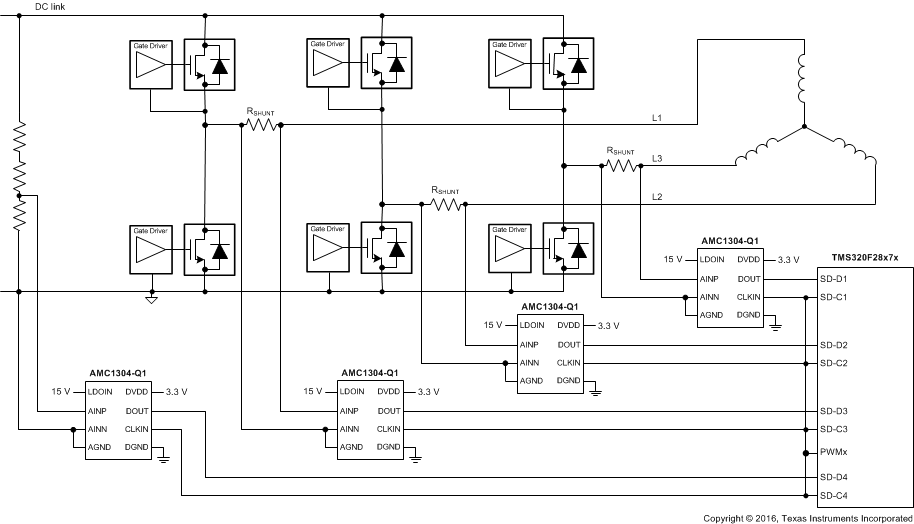Figure 54. The AMC1304-Q1 in a Traction Inverter Application

#### 9.2.1.1 Design Requirements

A typical operation of the device in a traction inverter application is shown in Figure 54. When the inverter stage is part of a motor drive system, measurement of the motor phase current is done via the shunt resistors (RSHUNT). Depending on the system design, either all three or only two phase currents are sensed.

In this example, an additional fourth AMC1304-Q1 is used to support isolated voltage sensing of the dc link. This high voltage is reduced using a high-impedance resistive divider before being sensed by the device across a smaller resistor. The value of this resistor can degrade the performance of the measurement, as described in the Isolated Voltage Sensing section.

#### 9.2.1.2 Detailed Design Procedure

The typically recommended RC filter in front of a ΔΣ modulator to improve signal-to-noise performance of the signal path is not required for the AMC1304-Q1. By design, the input bandwidth of the analog front-end of the device is limited to 1 MHz.

For modulator output bit-stream filtering, a device from TI's TMS320F2807x family of low-cost microcontrollers (MCUs) or TMS320F2837x family of dual-core MCUs is recommended. These families support up to eight channels of dedicated hardwired filter structures that significantly simplify system level design by offering two filtering paths per channel: one providing high accuracy results for the control loop and one fast response path for overcurrent detection.

#### 9.2.1.3 Application Curve

In motor control applications, a very fast response time for overcurrent detection is required. The time for fully settling the filter in case of a step-signal at the input of the modulator depends on its order; that is, a sinc3 filter requires three data updates for full settling (with fDATA = fCLK / OSR). Therefore, for overcurrent protection, filter types other than sinc3 can be a better choice; an alternative is the sinc2 filter. Figure 55 compares the settling times of different filter orders.

The delay time of the sinc filter with a continuous signal is half of its settling time.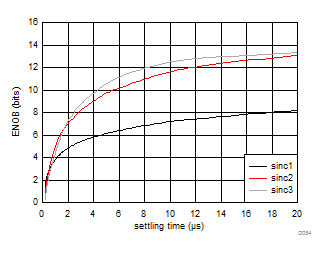Figure 55. Measured Effective Number of Bits versus Settling Time

#### 9.2.2 Isolated Voltage Sensing

The AMC1304-Q1 is optimized for usage in current-sensing applications using low-impedance shunts. However, the device can also be used in isolated voltage-sensing applications if the affect of the (usually higher) impedance of the resistor used in this case is considered.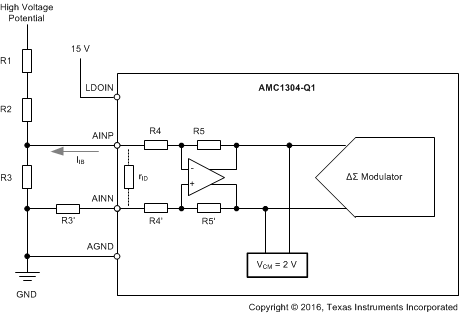Figure 56. Using the AMC1304-Q1 for Isolated Voltage Sensing

#### 9.2.2.1 Design Requirements

Figure 56 shows a simplified circuit typically used in high-voltage-sensing applications. The high impedance resistors (R1 and R2) are used as voltage dividers and dominate the current value definition. The resistance of the sensing resistor R3 is chosen to meet the input voltage range of the AMC1304-Q1. This resistor and the differential input impedance of the device (the AMC1304x25-Q1 is 25 kΩ, the AMC1304x05-Q1 is 5 kΩ) also create a voltage divider that results in an additional gain error. With the assumption of R1, R2, and RIN having a considerably higher value than R3, the resulting total gain error can be estimated using Equation 4, with EG being the gain error of the AMC1304-Q1.

Equation 4.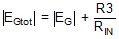This gain error can be easily minimized during the initial system-level gain calibration procedure.

#### 9.2.2.2 Detailed Design Procedure

As indicated in Figure 56, the output of the integrated differential amplifier is internally biased to a common-mode voltage of 2 V. This voltage results in a bias current IIB through the resistive network R4 and R5 (or R4' and R5') used for setting the gain of the amplifier. The value range of this current is specified in the Electrical Characteristics table. This bias current generates additional offset error that depends on the value of the resistor R3. Because the value of this bias current depends on the actual common-mode amplitude of the input signal (as illustrated in Figure 57), the initial system offset calibration does not minimize its effect. Therefore, in systems with high accuracy requirements, TI recommends using a series resistor at the negative input (AINN) of the AMC1304-Q1 with a value equal to the shunt resistor R3 (that is, R3' = R3 in Figure 56) to eliminate the affect of the bias current.

This additional series resistor (R3') influences the gain error of the circuit. The effect can be calculated using Equation 5 with R5 = R5' = 50 kΩ and R4 = R4' = 2.5 kΩ (for the AMC1304x05-Q1) or 12.5 kΩ (for the AMC1304x25-Q1).

Equation 5.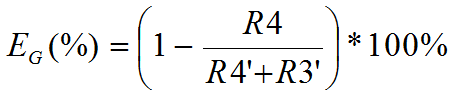#### 9.2.2.3 Application Curve

Figure 57 shows the dependency of the input bias current on the common-mode voltage at the input of the AMC1304-Q1.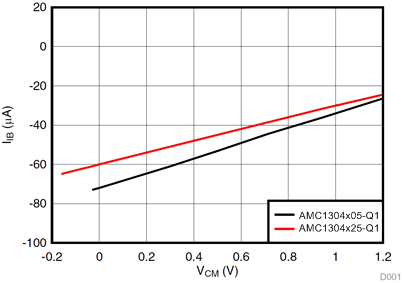Figure 57. Input Current vs Input Common-Mode Voltage

#### 9.2.3 Do's and Don'ts

Do not leave the inputs of the AMC1304-Q1 unconnected (floating) when the device is powered up. If both modulator inputs are left floating, the input bias current drives them to the output common-mode of the analog front end of approximately 2 V that is above the specified input common-mode range. As a result, the front gain diminishes and the modulator outputs a bitstream resembling a zero input differential voltage.